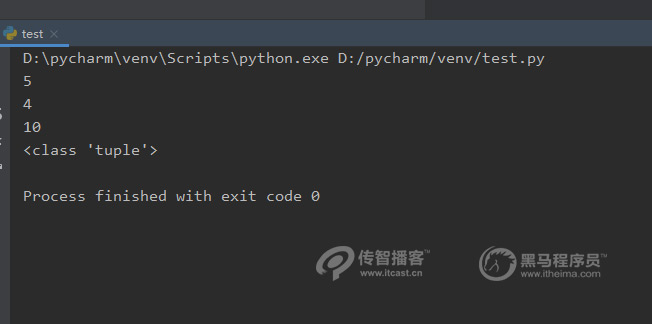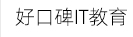### 全国中心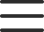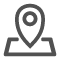# Python元组介绍：10分钟掌握元组用法

Python的元组(tuple)与列表类似，不同之处在于元组的元素不能修改，元组使用圆括号包含元素，而列表使用方括号包含元素。元组的创建很简单，只需要在圆括号中添加元素，并使用逗号分隔即可，示例代码如下：

tuple_one = ("java", "python", "PHP", "C++")
print(tuple_one)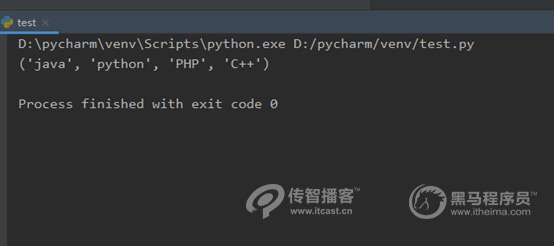1、访问元组

tuple_one = ("java", "python", "PHP", "C++")
print(tuple_one)
print(tuple_one)
print(tuple_one)
print(tuple_one)1、元组数据不能修改

tuple_one = ("java", "python", "PHP", "C++")
tuple_two = ("1", "2", "3")
tuple_three = tuple_one + tuple_two
print(tuple_three)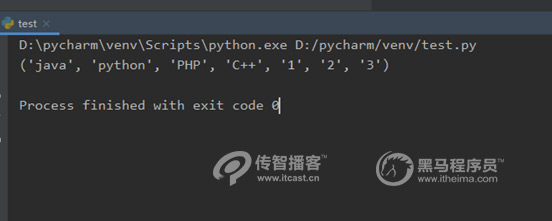tuple_one = ("java", "python", "PHP", "C++")
#把元组中的第一个元素修改为“传智播客”
tuple_one = "传智播客"
print(tuple_one)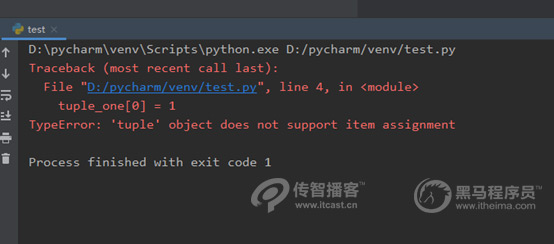2、遍历元组

tuple_one = ("java", "python", "PHP", "C++", "学IT到传智播客")

for subject in tuple_one:
#注意for循环后的print()语句的缩进，不然会报错
print(subject)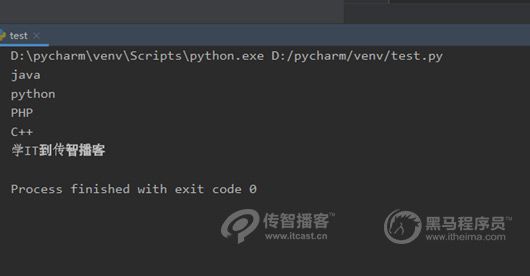3、内置元组函数哪些？

len(tuple) 表示计算元祖

max(tuple) 返回元祖中的元素最大值

min(tuple) 返回元组中元素最小值

tuple(seq)  将列表转为元组

tuple_suject = ("java", "python", "PHP", "C++", "学IT到传智播客")
# 计算元组中元素最大值和最小值
len_size = len(tuple_suject)
print(len_size)
# 返回元组元素最大值和最小值
tuple_number = ("4", "10", "16", "19", "2")
max_number = max(tuple_number)
min_number = min(tuple_number)
print(max_number)
print(min_number)
# 将列表转为元组
list_subject = ["java", "python", "大数据", "软件测试", "PHP"]
tuple_subject2 = tuple(list_subject)
# 打印tuple_subject2数据类型
print(type(tuple_subject2))